# How much of an object can an observer see

In the following diagram how much of the arrow can an observer see.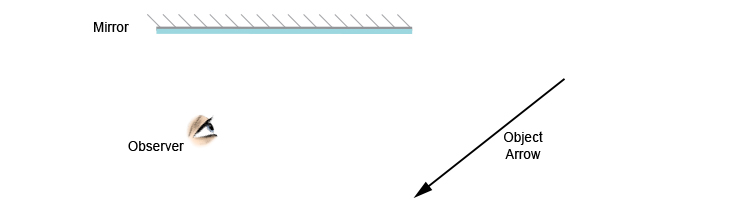To work this out we need to apply the law of reflection. The first stage of this is to draw an image behind the mirror by marking either end of the image as being equal-distance and perpendicular (at 90°) from the mirror.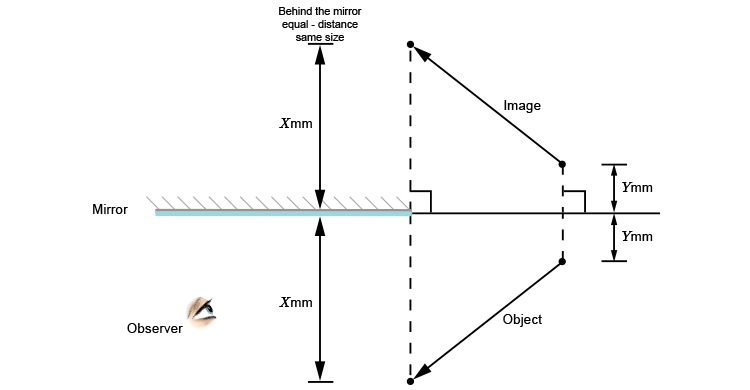We end up with a diagram as follows: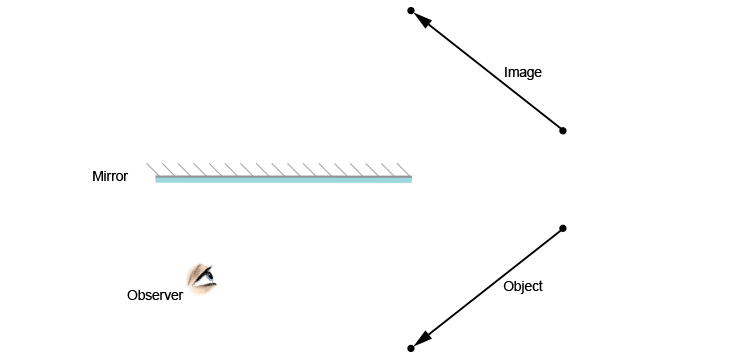The second stage is to draw a ray to the observer from the arrow end of the image and then join the reflected light ray from the mirror to the arrow end of the object.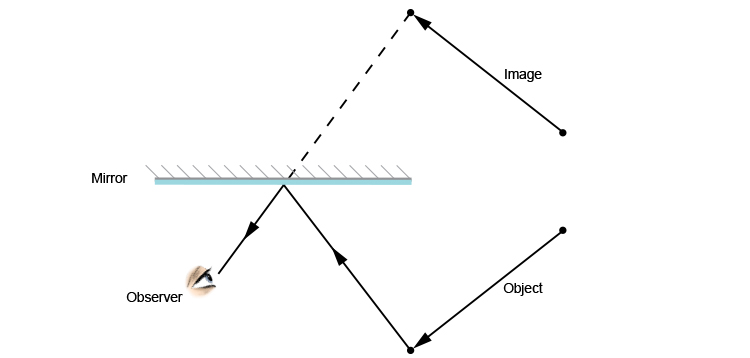Because the object is one continual line with an arrow at the end, the only way to find out how much of the line can be seen by the observer, you must reflect off the edge of the mirror, working back from the observer.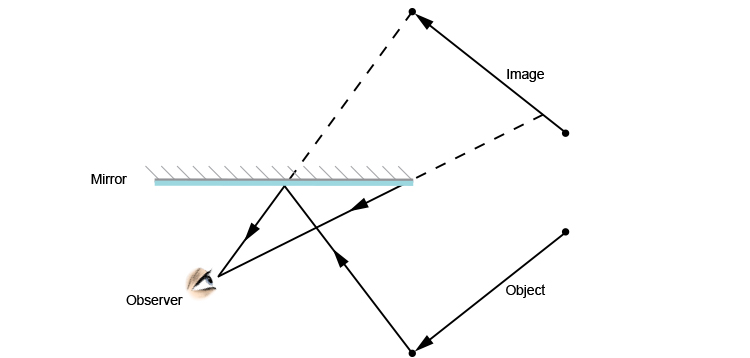Use the rule, "The angle of incidence equals the angle of reflection". We can draw a normal line and use a protractor to work out where the ray of light came from on the object.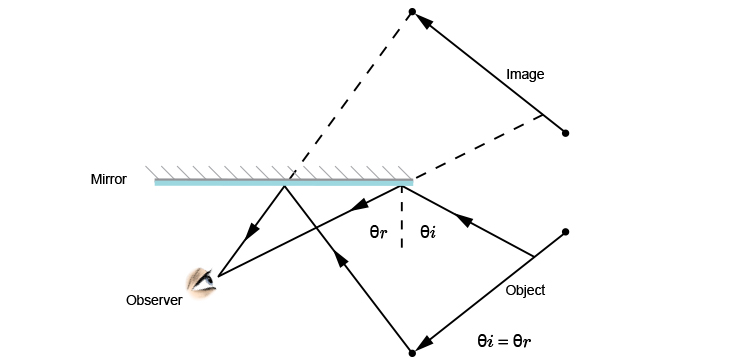We can now show you what part of the object the observer can see reflected in the mirror.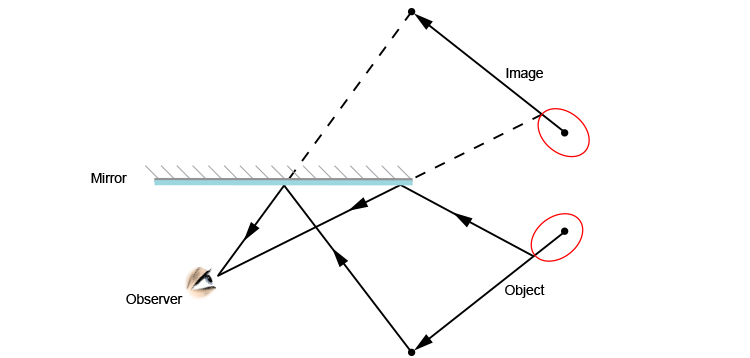The part circled in red cannot be seen by the observer.

## Conclusion

Using a ray diagram is useful in showing why only a portion of the image of an object can be seen from a given location.